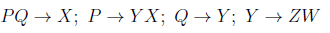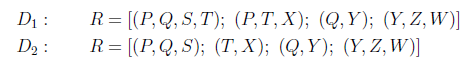Related Articles

# GATE | GATE CS 2021 | Set 1 | Question 43

• Last Updated : 24 May, 2021

Consider the relation R(P,Q,S,T,X,Y,Z,W) with the following functional dependencies.Consider the decomposition of the relation R into the constituent relations according to the following two decomposition schemes.Which one of the following options is correct?
(A) D1 is a lossless decomposition, but D2 is a lossy decomposition
(B) D1 is a lossy decomposition, but D2 is a lossless decomposition
(C) Both D1 and D2 are lossless decompositions
(D) Both D1 and D2 are lossy decompositions

Explanation: Lossless-Join Decomposition:
Lossless-Join Decomposition:
Decomposition of R into R1, R2, R3, R4 is a lossless-join decomposition if at least one of the following functional dependencies are in F+ (Closure of functional dependencies):

```R1 ∩ R2 → R1
OR
R1 ∩ R2 → R2```

For decomposition D1:

R1(PQST)

R2(PTX)

R3(QY)

R4(YZW)

R1 ∩ R2 = (PT)+ = PTYXZW , it is a super key, so we can merge R1 and R2.

combined table T1 is PQSTX

similarly,

R3 ∩ R4 =(Y)+ = YZW, it is a super key,  so we can merge R3 and R4.

another combined table T2 is QYZW.

now, Q is common in both T1 and T2.

T1 ∩ T2 = Q+ = QYZW, it is a super key, so we can merge T1 and T2.

after combining, we get original table PQSTXYZW,

Hence D1 is lossless join decomposition.

For decomposition D2:

R1(PQS)

R2(TX)

R3(QY)

R4(YZW)

since R2 has no common attributes as the primary key, so R2 cannot be merge with any other table,

Hence D2 is lossy decomposition.

Attention reader! Don’t stop learning now.  Practice GATE exam well before the actual exam with the subject-wise and overall quizzes available in GATE Test Series Course.

Learn all GATE CS concepts with Free Live Classes on our youtube channel.

My Personal Notes arrow_drop_up